## Journal of Chemistry and Applied Chemical EngineeringISSN : 2576-3954

All submissions of the EM system will be redirected to Online Manuscript Submission System. Authors are requested to submit articles directly to Online Manuscript Submission System of respective journal.

Research Article, J Chem Appl Chem Eng Vol: 2 Issue: 1

# The Simms Constants as Parameters in Hyperbolic Functions Related to Acid-Base Titration Curves

Anna Maria Michalowska-Kaczmarczyk1, Aneta Spórna-Kucab2, Agustin Garcia Asuero3 and Tadeusz Michalowski2*

1Department of Oncology, The University Hospital in Cracow, 31-501 Cracow, Poland

2Department of Analytical Chemistry, Technical University of Cracow, 31-155 Cracow, Poland

3Department of Analytical Chemistry, The University of Seville, 41012 Seville, Spain

Faculty of Chemical Engineering and Technology, Cracow University of Technology, Warszawska 24, 31-155 Cracow, Poland
Tel:
+48126282035
E-mail:
michalot@o2.pl

Received: January 01, 2018 Accepted: January 01, 2018 Published: February 02, 2018

Citation: MichaÅowska-Kaczmarczyk AM, Spórna-Kucab A, Asuero AG, MichaÅowski T (2018) The Simms Constants as Parameters in Hyperbolic Functions Related to Acid-Base Titration Curves. J Chem Appl Chem Eng 2:1. doi: 10.4172/2576-3954.1000111

## Abstract

The Simms constants (gi) are parameters of transformed equations for acid-base titration curves, obtained from rational functions of the Padé type. The relationships between gi and successive dissociation constants Ki values for polyprotic acids are formulated. The models related to acid-base titration curves are expressed in terms of hyperbolic functions. Some relations of gi to the Fermi- Dirac distribution function are indicated.

## Notations

D – titrand, gi – Simms constant; T – titrant

## Introduction

The term ‘Simms constants’ is an eponym related to virtual equilibrium constants (gi) suggested by Simms [1-5], known also as ‘titration indices’ or ‘titration constants’ [6,7]. The gi were considered first in biological context [8-10], and later in a series of papers involved with titrimetric methods of analysis [11-15]. In particular, the gi concept can be applied in modeling the equilibria occurred in complex acid-base systems, where the isomolarity condition was fulfilled [16,17]. Later on, the Simms constants were applied to modeling of titration curves perceived from the viewpoint of total alkalinity (TAL) [18-20], also with fulvic acids (FA) involved . The Simms can be considered [16-24] in context of rational functions of the Padé type , with activity h of H+1 ions as the variable.

Application of the Simms constants enables any q-protic acid HnL (C0 mol/L), characterized by successive dissociation constants (Ki) values [10,16], to be considered as a mixture of q weak monoprotic acids HL(k) (k = 1, …,q) of the same concentration, i.e., C0 mol/L; the Simms constants gi are ascribed to these acids as (virtual) dissociation constants. The relations between gi and Ki values were formulated. Mathematical transformations made for this purpose resemble the technique called as decomposition of rational functions into a sum of partial fractions, well-known to students during the course in mathematical analysis (integral calculus), see e.g. . The Simms constants are involved in the partial fractions of this kind.

In this paper, the Simms constants (gi) will be referred to D+T systems, with MmHn-mL (C0) + HB (Ca) + MOH (Cb) solution, called as the sample tested (ST), see Table 1. Depending on the pre-assumed composition of the species formed in a system, we consider first the more general case where complexes of MaHiBbL+a+i-b-n type are formed; a, b = 0,1,…, i.e., the species HiL+i-n (i=0,…,q) are also admitted here (at a=b=0). The D+T system where only the species HiL+i-n (i=0,…, q) are formed, is considered as a particular case of the more complex system of the species. The interrelations where hyperbolic functions are involved with parameters of this simpler system, with dissociation constants (Ki) or stability constants of proto-complexes (KiH), known from tables of equilibrium data, are presented here. Stability constants of the mixed complexes are rarely met in literature; see e.g. [27-30].

System no. ST HB (CB) MOH (CM) MB (CMB) F
D T D T D T D T
1 VST VST VB VMB V*MB2 VST VST VB V*MB VMB3 VST VST VM VMB V*MB4 VST VST VM V*MB V*MBTable 1: Composition of titrand (D) and titrant (T) for different isomolar systems and the related expressions for F (Eq. 6); W = V0+V.

## Composition of titrand (D) and titrant (T)

The D and T are prepared in volumetric flasks: F1 and F2, each with a volume of Vf mL. First, equal volumes VST of a sample tested composed of MmHn-mL (C0) + HB (Ca) + MOH (Cb) are introduced into F1 and F2. In turn, VB mL of HB (C) or VM mL of MOH (C) is added into F1. Then V*MB mL of MB (CMB) is introduced into F1, and VMB mL of MB (CMB) is introduced into F2. The volumes V*MB and VMB of MB fulfill the optional relations:(1)

(see Table 1). Both flasks are then supplemented with distilled water to the mark, and mixed thoroughly.

The volume V0 mL (V0 ≤ Vf) is taken for analysis and titrated as D with T, added in portions; V mL is the total volume of T added from the beginning of the titration to a given point of the titration. The value

W = V0+V (2)

is the total volume of D+T system, at a given point of titration.

## Formulation of D+T system

Denoting [MaHiBbL+a+i-b-n ] =caib for brevity, and applying the notations:(3)(4)(5)we get the relation(6)

(see Table 1). The relations for F in the systems 2 – 4 are obtained similarly. (Equation 6) can be transformed into the form(7)

Note that(8)

where :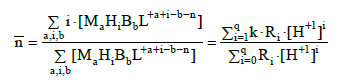(9)(10)(11)

Applying (8) in (7), we have(12)

where [18-20,30,31]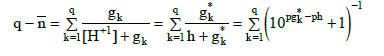(13)

## A Simpler System

The simpler case is the system, where the species HiL+i-n (i=0,…,q) are formed in the D+T mixture. These species can be characterized, optionally, by (successive) dissociation constants, Kj (j = 1,…,q):(14)

or by stability constants KiH of the related proto-complexes, HiL+i-n,(15)

Then we get the relations(16)

Applying (15) in the relation(17)

we have(18)

The Simms constants gk are interrelated with successive dissociation constants Kj (Equation 14) of the acid HnL considered; we have a set of interrelations:The expression for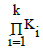(in Equations 19), formulated for q-protic acid, is a sum involving(20)

components  formed from k different gi values. In particular, for H3PO4 (acid of H3L type, q=n=3):

at k=1 we have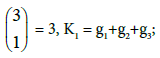at k=2 we have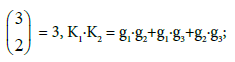at k=3 we haveGeneralizing, the binomial coefficient(Equation 20) expresses the number of distinct k-element subsets, formed from a set containing q different elements , as in the Pascal’s triangle .

The gi values can be calculated, provided that KiH (Equation 16) or Ki (Equation 14) values are known beforehand. Such calculations can be done with use of the iterative computer programs , as one specified in . In particular,

• for H2CO3 (q = 2): pK1 = 6.3, pK2 = 10.1, we have: pg1 = 6.300069, pg2 = 10.099931;

• for H3PO4 (q = 3): pK1 = 2.1, pK2 = 7.2, pK3 = 12.3, we have: pg1 = 2.100003, pg2 = 7.200000, pg3 = 12.299997.

As we see, pgi ≈ pKi values are here not distant from values. The differences | pgi – pKi | are greater when pKi values for a polyprotic acid are closer to each other.

For comparative purposes, we consider V0 mL of titrand D containing a mixture of q weak monoprotic (qk=nk=1) acids HL(k) (C0k; k=1,…,q), titrated with V mL of (a) HB (C) or (b) MOH (C) as T, we get the equations:(21)

and then(22)

where:the related hybrid dissociation constants,Then we have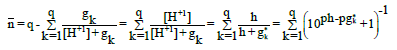(23)

## The Relative Contents of the Components Constituting D and T

The D and T include sample tested, ST (Table 1). If CMB >> Σa,i,b caib then [M+1] and [B-1] values are practically constant during the titration. Moreover, we assume. Similar composition of D and T guarantees the stability of ionic strength of the solution. The relative permittivity ε is also kept constant if the D and T compositions are similar; it makes also the volumes additivity more accurate than when mixing various aqueous solutions. Then the isomolarity condition (Equation 1) enables to keep approximately constant values of the equilibrium constants, under isothermal conditions.

Therefore, the values of Ri (in Equation 10) or Ki (in Equation 18) are practically constant during the titration carried out under such conditions. The hydrogen ion activity coefficient γ = γH+1 has also a stable value. The titration in isomolar systems makes it possible to determine γH+1 as one of the physicochemical parameters of the system, along with other equilibrium constants values .

## Formulation in terms of Hyperbolic Functions

The related formulas can be expressed in terms of hyperbolic functions . For this purpose, we denote: z = ln10âpH, w = ln10âpKW, sk = log10âpgk. Then applying the identity(24)

we get:(25(26)

From Equation 8, 13, 26 we have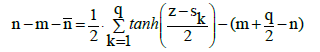(27)

The formulas involved with ph and gk* look alike. From Equations: 12, 13, 25 and 26, for C0k = C0, we get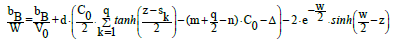(28)

The hyperbolic functions can also be applied to more complex acid-base systems, discussed in [18-20,37].

## Rational Functions

The general form of a rational function of variable x , y = y(x), is the quotient of polynomials p(x) and q(x), i.e.(29)

where m ≥ 1, i.e., the denominator q(x) involves explicitly the variable x.

The titration curves related to isomolar systems can be presented in the form of rational functions of the Padé type [16,17,20-24]. For example, for the mixture HL (C0) + HB (Ca) applied as ST in the system 3, we obtain the function(30)

where: A1 = â KWâx0γ3 ; A2 = bMâx0âγ2 ; A3 = (KW + dâ(C0 + Ca – aM/ V0)âx0)âγ2; A4 = bMâγ ; A5 = (dâ(Ca – aM/V0) – x0)âγ, aM = bM/d; x0 = 1/ K1, where [H+1][L-1] = K1â[HL]; the activity of hydrogen ions, x = h, is the variable in Eq. 30.

Special cases of rational functions are Möbius transformations . The rational functions were also applied in different methods of chemical analysis, namely: in modified Gran methods of titrimetric analyses [39-43], for calibration curve, and standard addition methods [44-47].

## Acid-base Micro-Equilibria as Emanation of Stochastic Processes

On the basis of formulation with the Simms constants involved one can state that the dissociation of H+1 from different protonation sites/centers proceeds independently, and the proton uptake/ dissociation from/to these sites (basicity centers) can be perceived as a stochastic process, categorized in terms of a success/failure. The degree of dissociation HL(k) = H+1 + L(k)-1 from the k-th site is(31)

where β = ln10. The αi = αi(pH) fulfills the properties of cumulative distribution function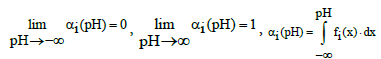(32)

where y = fi(pH) is the probability density function(33)

It implies that(34)

The function (34) plotted in Figure 1 appears some similarities with the Fermi-Dirac distribution function .

Figure 1: The fipH) vs. pH relationships (Eq. 34) plotted for pgk = 5.0.

The paper presents formulation of acid-base titration curves of different degree of complexity, for the D+T systems prepared according to unconventional mode, where D and T are prepared in accordance with the principle of isomolar solutions, suggested and formulated first time in the papers [16,17,21-24]. This procedure, where D and T have similar composition, secures constancy of equilibrium constants, activity coefficient of H+1 ions, and relative permittivity of D+T system during the titration performed under isothermal conditions.

Assuming formation of the species of (i) HiL+i-n or (ii) MaHiBbL+a+ib-n type (i = 0,…,q) formed by an acid HnL in the system where the species M+1 and B-1 are also involved, the relations involving the mean number of protons, Ín (Eq. 9 or 18) attached to the basic form L-n and the Simms constants gk were formulated. The partial ratios involved with gk were expressed in terms of the hyperbolic tangent (tanh) functions. The α (Eq. 4) was expressed in terms of hyperbolic sine (sinh). The partial ratios (Eq. 26) have the form (Eq. 34) similar to the one related to the density function in the Fermi-Dirac distribution function. Moreover, in , the inverse hyperbolic function argsinh  was applied for titration curve related to argentometric titration, and inverse hyperbolic function argcosh  as applied [28-30,52] for other titrations.

It were also proved that the titration of HnL (C0) with MOH is equivalent to titration of the mixture of q monoprotic acids, HL(i) (C0), with the related dissociation constants gk = K1k = [H] [L(k)] /[ HL(k) . In other words, application of Simms constants principle provides a kind of ‘homogenization’, where polyprotic acids are transformed into the mixture of monoprotic acids. It is a very important property, especially advantageous when considered in context with titration of solutions whose composition and then acid-base properties are unknown a priori, e.g., fulvic acids. It particularly refers to determination of total alkalinity of natural (e.g. marine) waters, wastes and different beverages, made according to titrimetric mode (pH titration).

## References